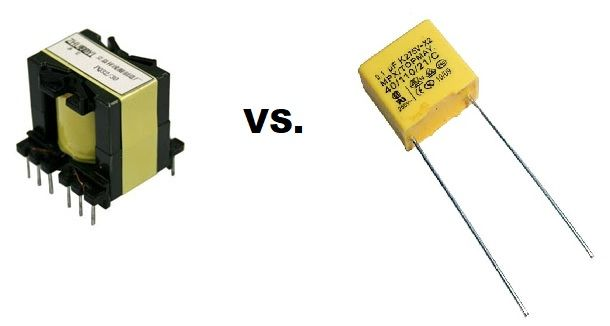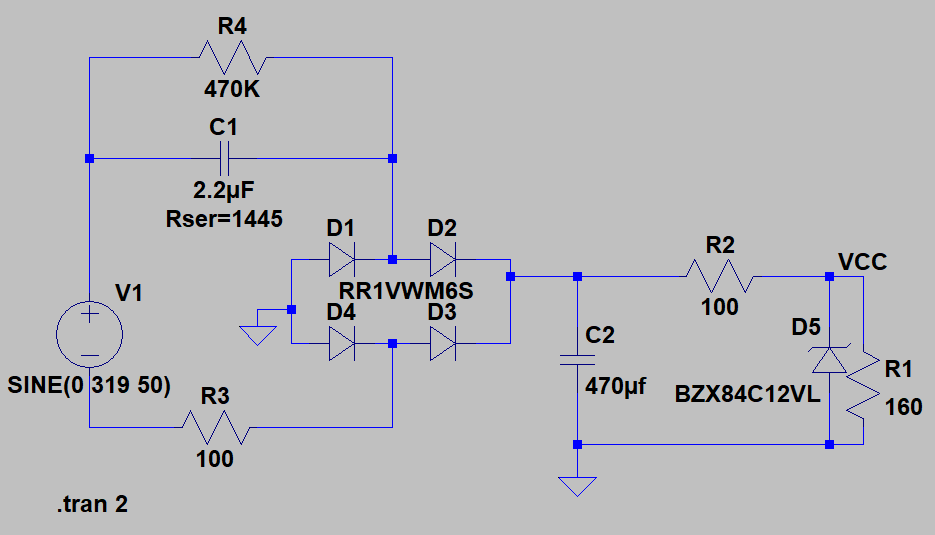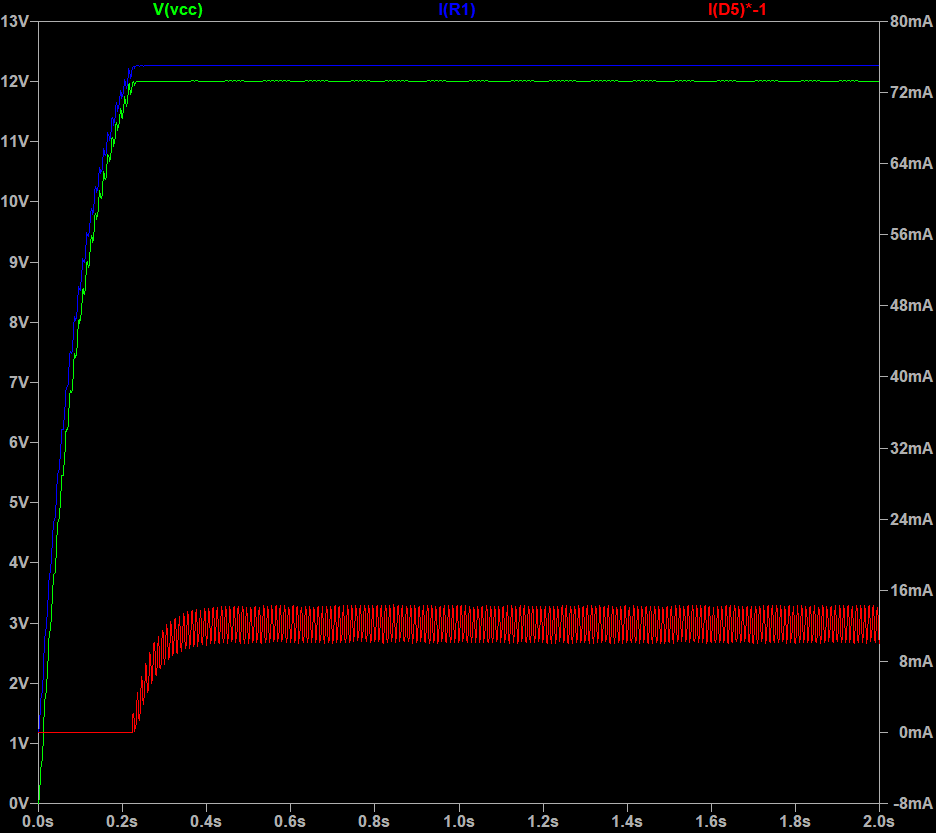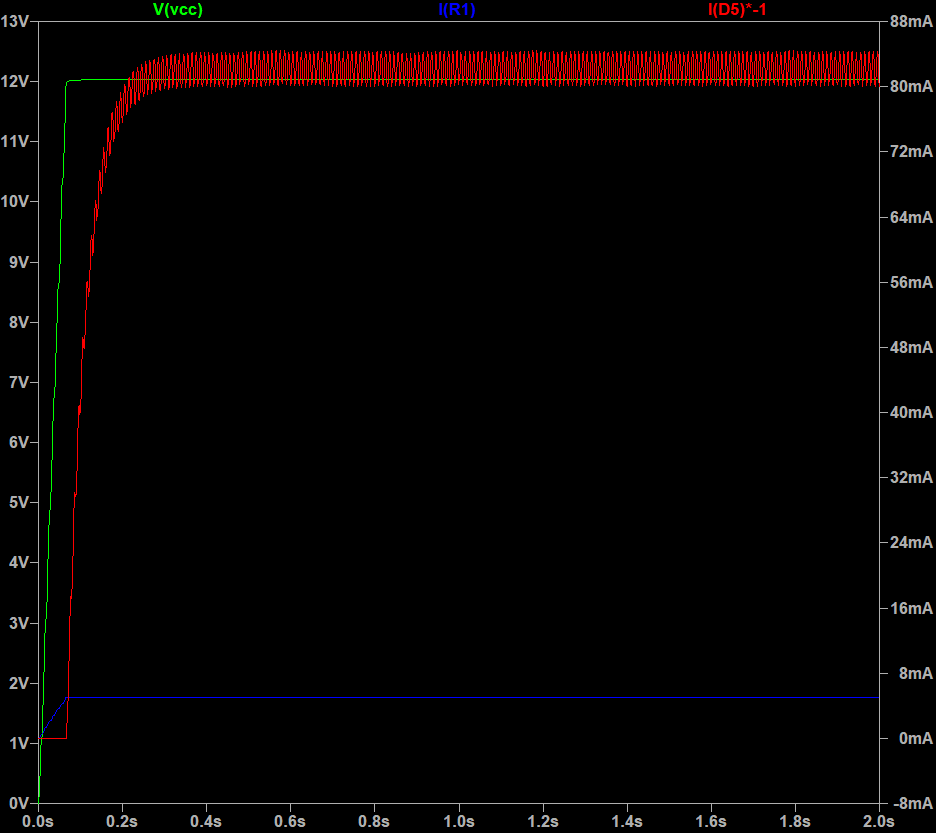# Transformerless PSU, is it worth it?When it comes to the design of a power supply that needs to convert AC mains voltage into a fixed DC voltage, it is the norm to think that a transformer is required to achieve that design goal. In this article, a different alternative will be presented, and more importantly, it will be simulated and compared to the standard transformer solution in terms of cost, size and performance.

## Theory

A popular way to step down a voltage used everywhere in low voltage/current applications is the voltage divider.

A transformerless power supply uses that principle to step down the voltage to the desired level, but instead of using a resistor it uses an X-rated capacitor which takes advantage of a property called reactance.

The reactance of a capacitor is the resistance value the capacitor will show in series for a particular frequency and capacitor value. Therefore, by choosing a capacitor value we can calculate the reactance with the formula:

Rx = 1/(2*pi*f*C)

As the circuit is connected to the AC mains, it is essential that an X-rated capacitor is used. An X-rated capacitor is specially designed to withstand high voltage spikes and to avoid a short circuit between the plates in case the capacitor breaks.

it is essential that an X-rated capacitor is used

Note that this article focuses on simulating the transformerless power supply and comparing it with a transformer PSU. If you want a more in-depth explanation of the theory behind please check this article at Hackaday and CircuitDigest.

## Transformerless PSU Design

The PSU will have the following design specs

• Step down and rectify 220VAC/50Hz into 12VDC
• The PSU must be able to source up to 75mA of current to the load

### Circuit

The following circuit topology was taken from the different consulted references:V1: The max amplitude is 220V*SRQT(2) at 50Hz

R4: bleeding resistor to discharge the capacitor when the AC connection is removed

R3 and R2: current limiting resistors

D1-D4: Discrete full bridge rectifier to convert the AC waveform into a DC voltage

C2: bulk capacitor to smooth the output voltage of the rectifier

D5: 12V Zener diode to prevent a higher voltage to be fed to the load

If you would really like to understand how this circuit works, I suggest downloading LTSPice, the simulation file, and try changing the parameters of the components.

### Choosing the X-rated capacitor C1

In order to source enough current to the load, we need a theoretical reactance of:

Rx = 220V/0.075A

Rx = 2933.33ohm

Therefore, we need a capacitance of:

C = 1/(2*pi*50Hz*2933.33ohm)

C = 1.085uF

A value of 2.2uF will be selected for the simulation with a theoretical reactance of:

R = 1/(2*pi*50Hz*2.2uFohm)

R = 1445ohm

## Simulation

The above circuit was simulated using LTSpice with two different conditions, at max load and at low current (5mA). Three different points were probed: VCC (expected 12V), I(R1) which is the load current and the current going through the Zener diode I(D5).#### Low current (5mA)### Analysis of simulation

As we can see from the above graphs, the power supply is capable of delivering up to 75mA at 12V. However, the Zener diode and current limiting resistors R3 and R2 are constantly dissipating a considerable amount of heat in order to maintain a stable power supply.

Dz_pdiss = 12V*0.084A = 1W

R2_pdiss = 100ohms*(0.084+0.05)^2 =0.8W

R4_pdis =~ R2_pdiss

## Comparison with a standard transformer PSU

With the data obtained from the simulation, commercial components can be selected from a supplier in order to compare the cost and size of the two different solutions.

### Cost

Components that are present in both solutions will not be quoted, such as the bulk capacitor C2.

All prices are valid for the 22/08/18 on Digikey for 1000 units

#### Transformerless BOM

C1  – EMI SUPP MP X2 RAD 310VAC 2.2UFX2 – 0.73\$/unit

R2 – RES 100 OHM 1W 5% AXIAL– 0.023\$/unit

R3 – RES 100 OHM 1W 5% AXIAL– 0.023\$/unit

R4 – RES 470K OHM 1/2W 5% CF MINI – 0.01\$/unit

D5 – DIODE ZENER 12V 1.25W DO214AC – 0.11\$/unit

Total = 0.9\$/unit

#### Transformer BOM

T1 – XFRMR LAMINATED 2.4VA THRU HOLE – 2.7\$/unit

Total = 2.7\$/unit

### Space

Space on a PCB is a bit relative, as it depends how you place and in what side the components are the traces width and max component height. For this comparison, we will just sum the total area the components used in a 2D plane.

#### Transformerless

C1 = 26mm*13mm = 338mm2

R2 and R3 = (2.4*6.3)mm*2 = 30.24mm2

R4 = 2.3mm*6.5mm = 14.95mm2

D5 = 4.5mm*2.5mm = 11.25mm2

Total area = 395mm2

#### Transformer

T1 = 34.93mm*28.58mm = 1000mm2 = total area

### Performance

This section will analyze the trade-offs of the transformerless power supply compared to a transformer solution

#### Dissipation and efficiency

The transformerless circuit has some serious issues regarding dissipation and efficiency.

As calculated above, the different components including the resistors and the Zener diode can dissipate up to 1 watt each. Apart from the fact that the components will get hot constantly which will decrease their lifetime, particularly the Zener diode, we have the following situation in terms of efficiency:

Our load of 12V and 0.075A is consuming 0.9W, however, in order for the circuit to supply this current and voltage, it needs to dissipate at least 3 times the amount of power required by the circuit in other components (R2, R3 and D1)!

In comparison, the regular transformer will only have an efficiency of 90% to 95%.

## Conclusion

The following table summarizes the findings discussed above:

TransformerlessTransformer
Cost0.9\$/unit for 1000 units2.7\$/unit for 1000 units
Size395mm21000m2
PerformanceExcessive heat dissipation and poor efficiencyGood efficiency

As we can see from the table, the transformerless PSU is definitely cheaper and can be designed smaller and lighter than a transformer PSU.

However, it comes at a high cost in performance and efficiency as it is constantly dissipating a considerable amount of power.

Therefore the ideal application for a power supply like this could be on a device that operates at a low ambient temperature (below 25C) and has access to an ample amount of energy. A sensor placed somewhere in Iceland at a geothermal power generation plant? Could be.

Have you built a transformerless PSU before? Share your challenges and findings!

## References

Transformerless Power Supply – CircuitDigest

Posted in: Product Development

1.M Z Ahmed says:
1.Roberto Weiser says: